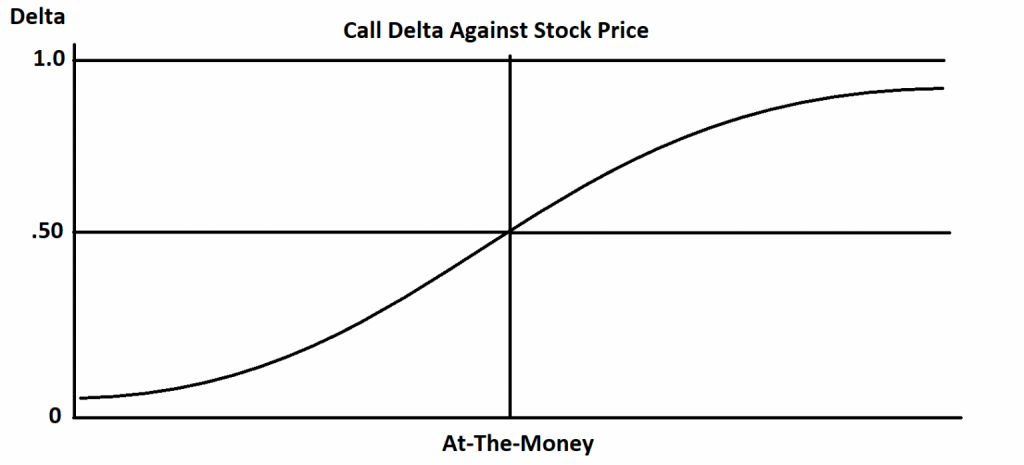# Delta of a StockThe delta of a stock is always 1.00 because delta is the variance of movement of a stock versus an option contract. The delta of an option is the magnitude of the move in the underlier that the option will capture currently based on the odds of the option expiring in-the-money. Delta quantifies the amount an option contract is exposed to moves in the price of the underlying asset.

Option delta is represented as the velocity of a price change in an option with a 1 point move in the underlying asset and is usually displayed as a decimal value. Delta values range between 0 and 1 for call options and -1 to 0 for put options.  Delta values are set in a range of a positive 1.0 to a negative –1.0, some express a .50 Delta by saying “50”.The delta of an option is the sensitivity of an option price to movement in relation to changes in the price of the underlying asset. It tells option traders how much the price of the option will change as the underlying stock or asset moves. A deep in-the-money option that is close to expiration will move nearly 100% dollar for dollar with its underlying stock when it is approximately a 1.00 delta. While an at-the-money option will have approximately a delta of .50, and will move 50% in step with its underlying stock. If your stock is at \$100 and you have a \$100 strike call option and the stock goes up \$2.00 your option with a .50 delta option contract will go up \$1.00. As an option gets deeper in-the-money and the odds of it expiring with intrinsic value increases so the delta expands in relation to these probabilities. An at-the-money option has 50/50 odds for directional movement and how it expires in relation to its strike price. As it gets deeper in-the-money the odds grow to 60%, 70%, 80% and at times 100% of it staying in-the-money on expiration.

Calls and puts have opposite deltas, call options are positive and put options are negative. A put option moves inversely to a call option.

Whenever you are long a call option, your delta will always be a positive number between 0 and 1. When the underlying asset increases in price, the value of your call option will also increase by the call options delta value. When the underlier price decreases the value of your call option will also decrease by the amount of the delta.

Put options have negative deltas, which will range between -1 and 0. When the underlier price increases the value of your put option will decreases by the amount of the delta value. When the price of the underlying asset decreases, the value of the put option will increase by the amount of the delta value.

Delta can be a good measure of the current odds of an option expiring in-the-money but those probabilities can change rapidly. An at-the-money option has 50/50 odds of it going either way by expiration and that is reflected in the delta of .50. However if you are buying a far out-of-the-money option with a delta of .10 that moves only one tenth in step with the underlying asset the odds are about 90% that your option will expire worthless. While 1 in 10 are terrible odds and if 9 losses all come in a row as the first nine option trades it could  blow up an account if the bets are too big. Option sellers like to write low delta option contracts to sell them short for a high probability of keeping the premium as a profit.

Options with .10 Delta can be looked at as lottery tickets with low odds of success. A 1.00 Delta option can be looked at as a replacement for stock but with less capital needed for the position. Delta shows you how much you make on a move and if your strike price is a good bet by expiration.

I created my Options 101 eCourse to give a new option traders a shortcut to a quick and easy way to learn how stock options work.